### Practice Set 5.2 Co-ordinate Geometry Class 10th Mathematics Part 2 MHB Solution

Practice Set 5.2

1. Find the coordinates of point P if P divides the line segment joining the points…
2. In each of the following examples find the co-ordinates of point A which divides…
3. Find the ratio in which point T(-1, 6)divides the line segment joining the pointsP(-3,…
4. Point P is the centre of the circle and AB is a diameter. Find the coordinates of point…
5. Find the ratio in which point P(k, 7) divides the segment joining A(8, 9) andB(1, 2).…
6. Find the coordinates of midpoint of the segment joining the points (22,20) and (0, 16).…
7. Find the centroids of the triangles whose vertices are given below. (1) (-7, 6), (2,…
8. In ΔABC, G (-4, -7) is the centroid. If A (-14, -19) and B(3, 5) then find…
9. A(h, -6), B(2, 3) and C(-6, k) are the co-ordinates of vertices of a triangle whose…
10. Find the co-ordinates of the points of trisection of the line segment AB with A(2, 7)…
11. If A (-14, -10), B(6, -2) is given, find the coordinates of the points which divide…
12. If A (20, 10), B(0, 20) are given, find the coordinates of the points which divide…

###### Practice Set 5.2
Question 1.

Find the coordinates of point P if P divides the line segment joining the points A(-1,7) and B(4,-3) in the ratio 2:3.

A point P(x,y) divides the line formed by points (a,b) and (c,d) in the ratio of m:n, then the coordinates of the point P is given by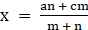and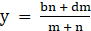In the given question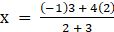x =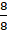= 1

y =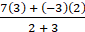y = 3

Hence the coordinates of the point are (1,3).

Question 2.

In each of the following examples find the co-ordinates of point A which divides segment PQ in the ratio a:b.

(1) P(-3, 7), Q(1, -4), a:b = 2:1

(2) P(-2, -5), Q(4, 3), a:b = 3:4

(3) P(2, 6), Q(-4, 1), a:b = 1:2

A point P(x,y) divides the line formed by points (a,b) and (c,d) in the ratio of m:n, then the coordinates of the point P is given byandWhere m and n is defined as the ratio in which the line segments are divided

1. x =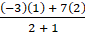x =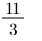y =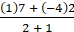y =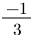2. x =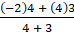X =y =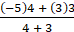y =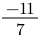3. x =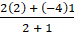= 0

y =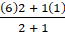y =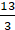Question 3.

Find the ratio in which point T(-1, 6)divides the line segment joining the pointsP(-3, 10) and Q(6, -8).

A point P(x,y) divides the line formed by points (a,b) and (c,d) in the ratio of m:n, then the coordinates of the point P is given byandIn the given question,

Let the point T divide the line PQ in the ratio m:n

Here x = -1 and y = 6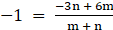...(1)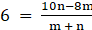.... (2)

Simplifying (1) we get,

-m-n = -3n + 6m

2n = 7m

Simplifying (2) we get,

6m + 6n = 10n-8m

14m = 4n

From both we get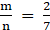Hence the point T divides PQ in the ratio 2:7

Question 4.

Point P is the centre of the circle and AB is a diameter. Find the coordinates ofpoint B if coordinates of point A and P are (2, -3) and (-2, 0) respectively.

According to the mid point theorem the coordinates of the point P(x,y) dividing the line formed by A(a,b) and B(c,d) is given by: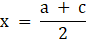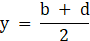In the given question A = (1,-3) and midpoint P is (-2,0).

Let coordinates of B be (c,d)

Then,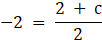And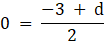Solving for c and d, we get

-4 = 2 + c

c = -6

d = 3

Hence the coordinates of point B are (-6,3).

Question 5.

Find the ratio in which point P(k, 7) divides the segment joining A(8, 9) andB(1, 2). Also find k.

A point P(x,y) divides the line formed by points (a,b) and (c,d) in the ratio of m:n, then the coordinates of the point P is given byandIn the given question,

Let the point P divide AB is the ratio 1:k

Y coordinate of P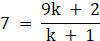Simplifying

7k + 7 = 9k + 2

2k = 5

k =and the ratio = 1:Therefore point P divides AB in the ratio 2:5

Question 6.

Find the coordinates of midpoint of the segment joining the points (22,20) and (0, 16).

According to the mid point theorem the coordinates of the point P(x,y) dividing the line formed by A(a,b) and B(c,d) is given by:The coordinates of midpoint(x,y) are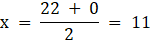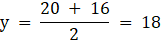Hence the coordinates are (11,18)

Question 7.

Find the centroids of the triangles whose vertices are given below.

(1) (-7, 6), (2, -2), (8, 5)

(2) (3, -5), (4, 3), (11, -4)

(3) (4, 7), (8, 4), (7, 11)

The coordinates of the centroid (x,y) od a triangle formed by points (a,b), (c,d), (e,f) is given by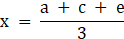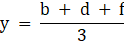1.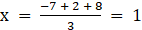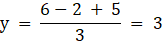2.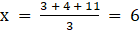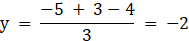3.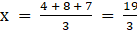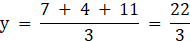Question 8.

In ΔABC, G (-4, -7) is the centroid. If A (-14, -19) and B(3, 5) then find theco-ordinates of C.

The coordinates of the centroid (x,y) od a triangle formed by points (a,b), (c,d), (e,f) is given byIn the given question (x,y) = (-4,-7)

Hence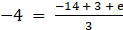Solving for e, we get

e = -1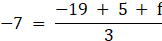Solving for f, we get

f = -7

Hence the coordinates of the third point are (-1,-7)

Question 9.

A(h, -6), B(2, 3) and C(-6, k) are the co-ordinates of vertices of a trianglewhose centroid is G (1, 5). Find h and k.

The coordinates of the centroid (x,y) od a triangle formed by points (a,b), (c,d), (e,f) is given byIn the given question: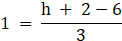Solving for h we get

h = 7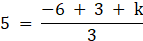Solving for k we get

k = 18

Question 10.

Find the co-ordinates of the points of trisection of the line segment AB with A(2, 7) and B(-4, -8).

let The points of trisection of a given line AB be P and Q

Then the ratio AP:PQ:QB = 1:1:1

Hence we get AP:PB = 1:2

And AQ:QB = 2:1

A point P(x,y) divides the line formed by points (a,b) and (c,d) in the ratio of m:n, then the coordinates of the point P is given byandTo find point P(x,y)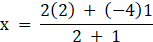x = 0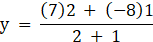y = 2

To find the point Q(x',y')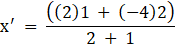x' = -2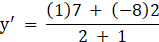y' = -3

Hence point P = (0,2) and Q = (-2,-3)

Question 11.

If A (-14, -10), B(6, -2) is given, find the coordinates of the points whichdivide segment AB into four equal parts.

let the points dividing AB be C,D,E.

AC:CD:DE:EB∷1:1:1:1

A point P(x,y) divides the line formed by points (a,b) and (c,d) in the ratio of m:n, then the coordinates of the point P is given byandFor C m:n ∷ 1:3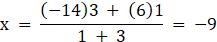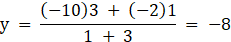For D m:n ∷2:2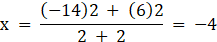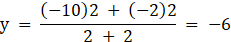For E m:n ∷ 3:1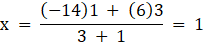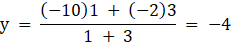Hence coordinates of C = (-9,-8)

D = (-4,-6)

E = (1,-4)

Question 12.

If A (20, 10), B(0, 20) are given, find the coordinates of the points which divide segment AB into five congruent parts.

Let the points dividing AB be C,D,E,F

AC:CD:DE:EF:FB∷1:1:1:1:1

A point P(x,y) divides the line formed by points (a,b) and (c,d) in the ratio of m:n, then the coordinates of the point P is given byandFor C m:n ∷ 1:4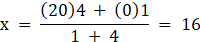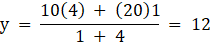For D m:n ∷ 2:3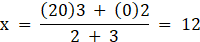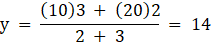For E m:n ∷ 3:2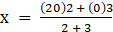= 8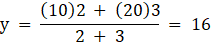For F m:n ∷ 4:1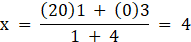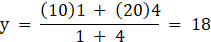## PDF FILE TO YOUR EMAIL IMMEDIATELY PURCHASE NOTES & PAPER SOLUTION. @ Rs. 50/- each (GST extra)

SUBJECTS

HINDI ENTIRE PAPER SOLUTION

MARATHI PAPER SOLUTION
SSC MATHS I PAPER SOLUTION
SSC MATHS II PAPER SOLUTION
SSC SCIENCE I PAPER SOLUTION
SSC SCIENCE II PAPER SOLUTION
SSC ENGLISH PAPER SOLUTION
SSC & HSC ENGLISH WRITING SKILL
HSC ACCOUNTS NOTES
HSC OCM NOTES
HSC ECONOMICS NOTES
HSC SECRETARIAL PRACTICE NOTES

2019 Board Paper Solution

HSC ENGLISH SET A 2019 21st February, 2019

HSC ENGLISH SET B 2019 21st February, 2019

HSC ENGLISH SET C 2019 21st February, 2019

HSC ENGLISH SET D 2019 21st February, 2019

SECRETARIAL PRACTICE (S.P) 2019 25th February, 2019

HSC XII PHYSICS 2019 25th February, 2019

CHEMISTRY XII HSC SOLUTION 27th, February, 2019

OCM PAPER SOLUTION 2019 27th, February, 2019

HSC MATHS PAPER SOLUTION COMMERCE, 2nd March, 2019

HSC MATHS PAPER SOLUTION SCIENCE 2nd, March, 2019

SSC ENGLISH STD 10 5TH MARCH, 2019.

HSC XII ACCOUNTS 2019 6th March, 2019

HSC XII BIOLOGY 2019 6TH March, 2019

HSC XII ECONOMICS 9Th March 2019

SSC Maths I March 2019 Solution 10th Standard11th, March, 2019

SSC MATHS II MARCH 2019 SOLUTION 10TH STD.13th March, 2019

SSC SCIENCE I MARCH 2019 SOLUTION 10TH STD. 15th March, 2019.

SSC SCIENCE II MARCH 2019 SOLUTION 10TH STD. 18th March, 2019.

SSC SOCIAL SCIENCE I MARCH 2019 SOLUTION20th March, 2019

SSC SOCIAL SCIENCE II MARCH 2019 SOLUTION, 22nd March, 2019

XII CBSE - BOARD - MARCH - 2019 ENGLISH - QP + SOLUTIONS, 2nd March, 2019

HSC Maharashtra Board Papers 2020

(Std 12th English Medium)

HSC ECONOMICS MARCH 2020

HSC OCM MARCH 2020

HSC ACCOUNTS MARCH 2020

HSC S.P. MARCH 2020

HSC ENGLISH MARCH 2020

HSC HINDI MARCH 2020

HSC MARATHI MARCH 2020

HSC MATHS MARCH 2020

SSC Maharashtra Board Papers 2020

(Std 10th English Medium)

English MARCH 2020

HindI MARCH 2020

Hindi (Composite) MARCH 2020

Marathi MARCH 2020

Mathematics (Paper 1) MARCH 2020

Mathematics (Paper 2) MARCH 2020

Sanskrit MARCH 2020

Important-formula

THANKS